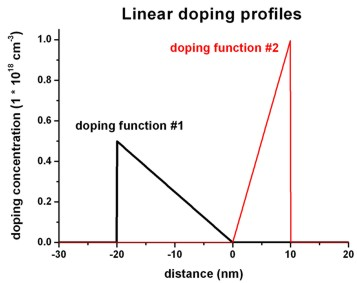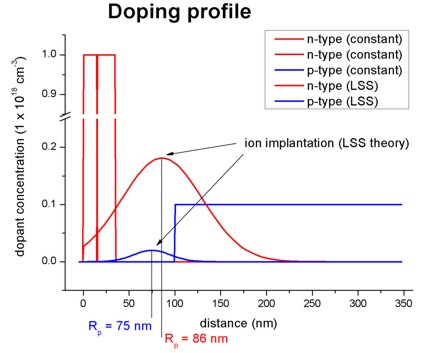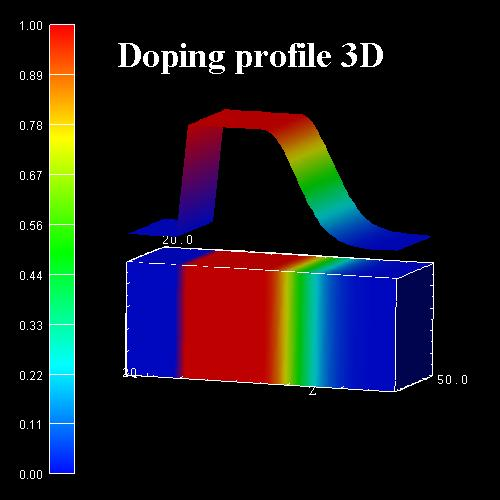nextnano.com  GUI: nextnanomat  Tool: nextnano++  Tool: nextnano³  Tool: nextnano.QCL  Download | Search | Copyright | News | Publications  * password protected nextnano³ softwareDoping function

# doping-function

## Doping profiles

Doping profiles can be specified by the product of` n ` functions.` n ` is the dimension of the simulation, i.e.` n `= 1, 2 or 3. Each function depends only on one coordinate.

The doping profile is independent of the regions specified before. The function is applied to the region given by the specifier` only-region`.

The doping concentration is at the position specified by the specifier``` position```.
The function is normalized such that the result is doping concentration at position` position`.
The impurity number specifies the kind of impurities used in the profile.

Note: Can be used with a doping concentration sweep where the` doping-concentration `is varied stepwise.

`!------------------------------------------------------------------!`
```\$doping-function                                        optional   !```
``` doping-function-number                 integer         required   !```
``` impurity-number                        integer         required   !```
``` base-function-1                        character       optional   !```
``` base-function-2                        character       optional   !```
``` base-function-3                        character       optional   !```
``` apply-function-1-along-dir             integer_array   optional   !```
``` apply-function-2-along-dir             integer_array   optional   !```
``` apply-function-3-along-dir             integer_array   optional   !```
``` doping-concentration                   double          required   !```
``` position                               double_array    optional   !```
``` parameters-base-function-1             double_array    optional   !```
``` parameters-base-function-2             double_array    optional   !```
``` parameters-base-function-3             double_array    optional   !```
``` exclude-materials                      integer_array   optional   !```
``` only-region                            double_array    optional   !                                                                    !  doping-profile-defined-by-function     character       optional   ! ``` new```                                                                    !  read-in-doping-file                    character       optional   !  doping-filename                        character       optional   !```
```                                                                   !  doping-sweep-active                    character       optional   !```
``` doping-sweep-step-size                 double          optional   !```
``` doping-sweep-number-of-steps           integer         optional   !```
```\$end_doping-function                                    optional   !```
`!------------------------------------------------------------------!`

Example

### Constant doping profile

``` \$doping-function   doping-function-number = 1   impurity-number        = 1               ! ```properties of this impurity type have to be here:` ` `\$impurity-parameters````   doping-concentration   = 0.5d0           ! 0.5 * 10^18 cm^-3   only-region            = 10.0d0 160.0d0  \$end_doping-function```

Note: Can be used with a doping concentration sweep where the` doping-concentration `is varied stepwise.

```doping-function-number = 1                        = 2                        = ...```
An integer number. At the very end, the doping function numbers must be given in a way, that a dense ascending series starting at` 1 ` can be formed.

```impurity-number = 1                 = 2                 = ...```
An integer number. Properties of this impurity number have to be specified later.
This is a reference to an impurity and its parameters which will be specified by``` \$impurity-parameters```.

### More complicated doping profiles

You can use different base functions along each simulation direction to define more complicated doping profiles.

`base-function-1 = string-1`
`base-function-2 = string-2`
```base-function-3 = string-3 ```a valid base function name
`string-i `can be one of the one-dimensional functions:``` constant```,``` linear```,``` gauss-1d```,``` step-1d```,``` well-1d```
The final doping profile will result from a product of these functions.

`parameters-base-function-1 = double1 ....`
`parameters-base-function-2 = double1 ....`
```parameters-base-function-3 = double1 .... ```function parameters
Parameters for the selected base functions. Dependent on the base function chosen, the following is expected:

### Constant base function: Constant doping profile

`base-function-1            = constant`
No further function parameters required in 1D simulations.

Note: Can be used with a doping concentration sweep where the` doping-concentration `is varied stepwise.

### Linear base function: Linear doping profile

```base-function-1            = linear```
The example shows which additional parameters are necessary.

Example:```\$doping-function  doping-function-number     = 1                       ! ```doping function #1```  impurity-number            = 1                       ! ```properties of this impurity type have to be specified below```  doping-concentration       = 0.5d0                   ! 0.5 * 1.0 * 10^18 cm^-3 = 0.5 * 10^18 cm^-3  position                   = -20d0                   ! ```doping concentration refers to that position, i.e. `-`20 nm```  only-region                = -20d0 0d0               ! ```only from `-`20 nm to 0 nm```  base-function-1            = linear                  ! ```linear doping profile```  apply-function-1-along-dir = 0 0 1                   ! ```along z direction```  parameters-base-function-1 = -20d0 0d0   0.5d0 0.0d0 ! (1) zmin = -20 nm      (2) zmax = 0 nm                                                       ! (3) 0.5 * 10^18 cm^-3  (4) 0.0 * 10^18 cm^-3  doping-function-number     = 2                       ! ```doping function #2```  impurity-number            = 2                       ! ```properties of this impurity type have to be specified below```  doping-concentration       = 1d0                     ! 1.0 * 10^18 cm^-3  position                   = 10d0                    ! ```doping concentration refers to that position, i.e. 10 nm```  only-region                = 0d0 10d0                ! ```only from 0 nm to 10 nm```  base-function-1            = linear                  ! ```linear doping profile```  apply-function-1-along-dir = 0 0 1                   ! ```along z direction```  parameters-base-function-1 = 0d0 10d0    0.0d0 1.0d0 ! (1) zmin = 0 nm         (2) zmax = 10 nm                                                       ! (3) 0.0 * 10^18 cm^-3   (4) 1.0 * 10^18 cm^-3 \$end_doping-function```

### LSS theory (Lindhard, Scharff, Schiott theory) - Gaussian distribution of ion implantation impurity profile

```base-function-1            = gauss-1d         ! ```LSS theory
```parameters-base-function-1 = center-coordinate  gauss-width         minimum-value  maximum-value center-coordinate ```is the position of the Gauss center along the relevant direction` i` in units of``` [nm]```.``` gauss-width ```is usually called sigma in the formula of the Gaussian distribution function (in units of` [nm]`).
For the meaning of``` gauss-width ```have a look at the 10 DM banknote of the German "Deutsche Mark" or any mathematical textbook.
` minimum-value `minimum  value of doping concentration in units of` 10``18`` [cm-3]`
` maximum-value `maximum value of doping concentration in units of` 10``18`` [cm-3]`

Within LSS theory the specifiers correspond to the following notations:
```base-function-1            = gauss-1d         ! ```LSS theory
```parameters-base-function-1 = projected-range    projected-straggle  minimum-value  maximum-value apply-function-1-along-dir = 0 0 1            ! ```along z direction``` ``` ```doping-concentration       = implanted-dose / ( SQRT(2*pi) * projected-straggle ) ! ```concentration at reference` position` (see below)``` position                   = projected-range  ! ```doping concentration refers to this position``` projected-range    ```Rp (ion's projected range) in units of` [nm]`, i.e. the depth where most ions stop.
`projected-straggle `Delta Rp (ion straggle) in units of` [nm]`, i.e. the statistical fluctuation of Rp. ``` ``` `implanted-dose``     ` phi in units of` [1/cm^2] `(dose of the implant), typical ranges are from` 1d11 `to``` 1d16```.``` ```The program calculates from these parameters the dopant distribution using LSS theory. The calculated dopant profile can be printed out using the keyword``` \$output-material ```and the specifier` doping-concentration`.
``` ```The 1D Schrödinger-Poisson tutorial shows a figure of two impurity profiles based on LSS theory.For further details see for example:
"Very brief Introduction to Ion Implantation for Semiconductor Manufacturing" by Gerhard Spitzlsperger

### User-defined doping function

Using the specifier` ```` doping-profile-defined-by-function```, one can define an arbitrary function n(x,y,z) for the doping profile.
The value of this function is finally multiplied by the value specified in``` doping-concentration```. Obviously, one can also set``` doping-concentration = 1d0```.
The variables x, y, z refer to the grid point coordinates of the simulation area in units of` [nm]`.

Example: The red Gaussian shaped curve in the above figure can also be achieved by defining the Gaussian function directly:

```\$doping-function  ...    %mu      = 86                                      !    mu ```of Gaussian distribution``` (DisplayUnit:nm)    %sigma   = 44                                      ! sigma ```of Gaussian distribution``` (DisplayUnit:nm)    %max_dop = 0.181337400182469                       ! ```maximum doping concentration at center of Gaussian distribution``` (DisplayUnit:1e18cm^-3)  doping-function-number             = 4               ! ```doping function #4```  impurity-number                    = 2               !  doping-concentration               = %max_dop        ! 1 * 10^18 cm^-3  doping-profile-defined-by-function = " exp(- (z-%mu)^2 / (2*%sigma^2) ) "  ! n(x,y,z) = ...   ```(dimensionless)```                                     = no              ! ```do not use user-defined doping function

The following operators and functions are supported:
```+ , - , * , / , ^ abs , exp , sqrt , log , log10 , sin , cos , tan , sinh , cosh , tanh , asin , acos , atan```

### Predefined doping functions

`base-function-1            = step-1d`
```parameters-base-function-1 = para(1) = center, para(2) = width, para(3) = leftval, para(4) = rightval```

`base-function-1            = well-1d`
```parameters-base-function-1 = para(1) = center, para(2) = width, para(3) = leftval, para(4) = rightval```
```                             para(5) = center, para(6) = width, para(7) = leftval, para(8) = rightval```
First 4 parameters for left step, second 4 parameters for right step.
This is a well with double gauss walls. The walls are centered at parameter``` center ``` and the slope of the walls is given by` width`.

`apply-function-1-along-dir = i j k`
`apply-function-2-along-dir = i j k`
`apply-function-3-along-dir = i j k`
Variation of` function-i ` is along the specified direction (```0 0 1 ```or``` 0 1 0 ```or` 1 0 0`).

```doping-concentration = double ```concentration at reference` position` (see below).
A doping concentration at the position specified by the next specifier (position). The function defined above is normalized such that the result is doping concentration at position` position`.

Example:
We take
a constant doping with a concentration 8.0*1018 cm-3.

1D simulation: 8.0d0 * 1018/cm3.
`   doping-concentration = `
`8.0d0`

2D simulation: 8.0d0 * 1018/cm3.
`   `
```doping-concentration = 8.0d0```

3D simulation: 8.0d0 * 1018/cm3
```   doping-concentration = 8.0d0```

So we take the value to be``` 8.0d0 ```because we assume a 3D doping although we do a 1D or 2D simulation.
Thus it would be wrong to take
- 2.0
*106 cm-1     in the 1D case (cubic root)
- 4.0
*1012 cm-2   in the 2D case (squared cubic root)

```position = coord1 ... ```Doping concentration refers to that position.
The coordinates of a position which is used to fix normalization of the doping function profile. Can be omitted only for constant doping.

```exclude-materials = num1 ... ```To keep certain materials free from doping (e.g. air).
A list of defined material numbers which should not be doped.

```only-region = coord1 ... ```Apply doping function only to this region (coordinates of a cube, rectangle, line).
Restrict doping to this region only. The region is either a cube, rectangle or a line. The coordinates given specify the extension of the region as usual.
Note: See comments on how to specify correct interfaces further below.

Example (2D):

```\$doping-function                                     !                                                      !  doping-function-number = 1                          !  impurity-number        = 1                          ! ```properties of this impurity type have to be specified by``` \$impurity-parameters  doping-concentration   = 10d0                       ! ```10 * 1018/cm3.```  only-region            = 0.0d0 50.0d0 30.0d0 60.0d0 ! xmin xmax  ymin ymax                                                      ! \$end_doping-function                                 !```

Note: It you want to generate a very "accurate" doping profile, then you should apply the doping between interfaces. Interfaces are set if the material number is different.
Example: You want to specify a doping between 35.0 and 35.3 nm. Then you should consider to define a separate region, a separate cluster and a separate material for this 0.3 nm region.

Accurate doping profile:
```   35.0     35.15    35.3                 ! [nm]```
```     x        x        x        x         ! ```
physical grid points```      |                 |                  ! ```interface```     o|o       o       o|o       o         ! ``` 'multiple grid point' grid points (including multiple points)``` ``````.         .       .         .             ! ```material grid points (material parameters)``` ----- _________________ ------------------! ```doping area```     0|1       1       1|0                 ! ```weighting factor```  ```

Not so accurate doping profile:
```   35.0     35.15    35.3                 ! [nm]```
```     x        x        x        x         ! ```
physical grid points```      |                                    ! ```no interface at``` 35.3 nm     o|o       o        o        o         ! ``` 'multiple grid point' grid points (including multiple points)``` ``````.         .       .         .             ! ```material grid points (material parameters)``` ----- ____________________ ---------------! ```doping area```     0|1       1       0.5                 ! ```weighting factor``` (an average)  ```

Another not so accurate doping profile:
```   35.0     35.15    35.3                 ! [nm]```
```     x        x        x        x         ! ```
physical grid points```                                           ! ```no interfaces at all```      o        o        o        o         ! ``` 'multiple grid point' grid points (including multiple points)``` ``````.         .       .         .             ! ```material grid points (material parameters)``` -- _______________________ ---------------! ```doping area```     0.5       1       0.5                 ! ```weighting factor```  ```

Obviously all three cases can produce different results.

Example (3D):

The following figure shows a 3D doping profile that is defined inside a 20 nm x 20 nm x 50 nm cube where the 50 nm are the z direction. The doping profile is homogeneous with respect to the (x,y) plane, it only varies along the z direction.The doping profile is constant between z = 10 nm and z = 25 nm with a concentration of 1 x 1018 cm-3. It has Gaussian shape from z = 25 nm to z = 45 nm. It is zero between z = 0 nm and z = 10 nm, as well as between z = 45 nm and z = 50 nm.

``` \$doping-function   !-------------------------------------   ! constant doping of 1 * 10^18 cm^-3.   !-------------------------------------   doping-function-number     = 1                                 ! ```first doping funtion```   impurity-number            = 1                                 !   doping-concentration       = 1.0d0                             ! 1.0d0 => 1.0 * 10^18 / cm^3   only-region                = 0d0 20d0  0d0 20d0  10.0d0 25.0d0 ! xmin xmax  ymin ymax  zmin zmax   !--------------------------------------------------------------------------   ! Gaussian shaped doping along z direction, constant doping in (x,y) plane   !--------------------------------------------------------------------------   doping-function-number     = 2                                 ! ```second doping function```   impurity-number            = 1                                 !   only-region                = 0d0 20d0  0d0 20d0  25.0d0 45.0d0 ! xmin xmax  ymin ymax  zmin zmax   base-function-1            = constant                          !   base-function-2            = constant                          !   base-function-3            = gauss-1d                          !   apply-function-1-along-dir = 1 0 0                             ! ```constant doping along x direction```   apply-function-2-along-dir = 0 1 0                             ! ```constant doping along y direction```   apply-function-3-along-dir = 0 0 1                             ! ```Gaussian shaped doping along z direction```   doping-concentration       = 1d0                               ! 1.0d0 => 1.0 * 10^18 / cm^3   position                   = 10d0 10d0 25d0                    ! ```doping concentration refers to that position```   parameters-base-function-1 = 0d0 100d0   parameters-base-function-2 = 0d0 100d0   parameters-base-function-3 = 25d0 6d0  0d0 1.0d0               ! center-coordinate  gauss-width  minimum-value  maximum-value  \$end_doping-function```

If you want to obtain the input file that was used to obtain this 3D doping profile plot (constant + Gaussian shape), please contact stefan.birner@nextnano.de.
`-> 3Ddoping_profile.in`

## Reading in doping profiles from a file

```read-in-doping-file = yes/no - ```flag for reading in doping profile from a file
```- ```valid for n-type and p-type doping
```- ```valid for arbitrary``` doping-function-number - ```can be combined with explicitly specifying doping profile by input file and by reading in doping profile from several files
`- `all doping functions will be added to the previously specified values (like superpositions)

Restrictions:
`- `The value of this profile is finally multiplied by``` doping-concentration```. Obviously, one can also set``` doping-concentration = 1d0```.
`- `You can specify a region (`only-region`) for which the doping file applies to, the outer region will not contribute.

`doping-filename = doping_input.dat`
```                = doping/doping_input.dat```
doping filename to be read in (e.g. experimental values). The string can include a folder name.
The ASCII file must contain 2 (1D), 3 (2D) or 4 (3D) columns in each line:

1D:``` x coordinate [nm]                                            doping concentration [1*1018 cm-3] ...                                                          ...```

2D:``` x coordinate [nm]    y coordinate [nm]                       doping concentration [1*1018 cm-3] ...                  ...                                     ...```

3D:``` x coordinate [nm]    y coordinate [nm]   z coordinate [nm]   doping concentration [1*1018 cm-3] ...                  ...                 ...                 ...```

The first line of this ASCII file can contain an optional header line with column descriptors.

If you want to obtain an input file that shows how to import a doping profile from a file
(`1D_read_in_potential_and_doping_profiles.in`,``` ``` `2D_read_in_potential_and_doping_profiles.in`,``` ``` `3D_read_in_potential_and_doping_profiles.in`),

## Doping concentration sweep

It is possible to sweep over the doping concentration, i.e. to vary the doping concentration stepwise.
In each doping sweep step, the specifier` doping-concentration `is increased by` doping-sweep-step-size `in units of` [1*1018 cm-3]`.
The output is labelled by` ..._ind000.dat`,` ..._ind001.dat`,``` ... ```for each doping sweep step.

``` doping-sweep-active           = yes       ! ```to switch on doping sweep```                                = no        ! ```to switch off doping sweep
``` doping-sweep-step-size        = 0.2d0     ! ```increase doping concentration in each step by ... in units of``` [1*1018 cm-3]                                            ! ```(This value can also be negative.)```  doping-sweep-number-of-steps  = 5         ! ```number of doping sweep steps

Restrictions:
`- `Voltage sweeps (`\$voltage-sweep`) and other sweeps cannot be combined with doping sweeps at present.
`- `Only one doping sweep is allowed at present.